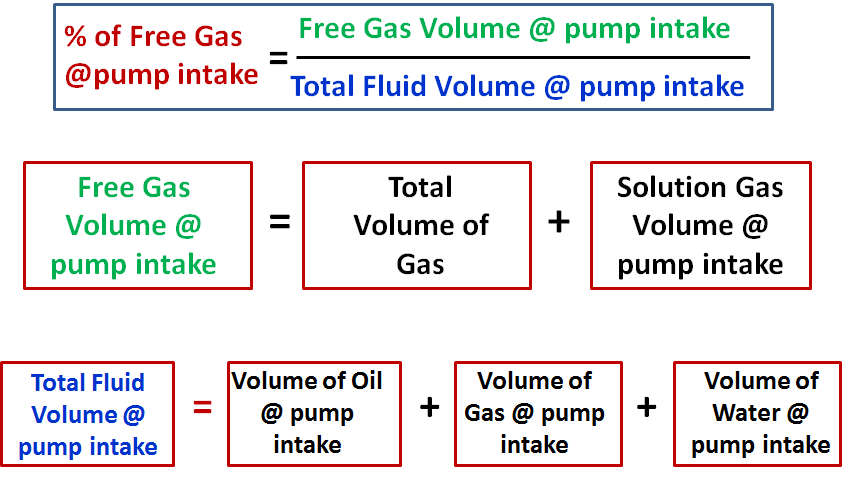# ESP design – Step 3: Gas Calculations

The presence of free gas in the tubing above the pump changes the fluid density, consequently reduces the required discharge pressure. Also the performance of centrifugal pumps is considerably affected by the presence of free gas in the pumped fluid. The pump starts producing lower than normal head as the produced GLR (Gas to Liquid Ratio) at the pumping conditions increases beyond a critical value. The critical value of the ratio or percentage of free gas present at the pump intake to the total volume of fluid depends on the pump impeller design (typical critical values are shown in the article “ESP: Gas handling device “). Therefore, it is essential to determine the percentage of free gas by volume at the pumping conditions in order select the proper pump and gas handling device (if required).

### Percentage of free gas by volume:

Assuming that Solution GOR (Rs), Gas Volume Factor (Bg) and Oil Formation Volume Factor (Bo) are known, the total volume of fluids and the percentage of free gas released at the pump intake should be calculated.Let’s start by determine the total volume of gas Vg:

Total Gas = (producing GOR x Oil rate)

[Oil rate]: stock tank barrels per day

The gas in solution at the pump intake can be determined as follows:

Solution Gas = (Solution GOR @ PIP x Oil rate) = (Rs x Oil rate)

PIP: Pump Intake Pressure

The free gas equals the total gas minus the solution gas:

Free Gas = Total Gas – Solution Gas

The volume of oil at the pump intake (Vo) equals stock tank barrels times oil formation volume factor (Bo):

Vo = Bo x BOPD

The volume of gas (Vg) at the pump intake equals the amount of free gas times gas volume factor (Bg):

Vg = Free Gas x Bg

The volume of water (Vw) at the pump intake is the same as stock tank barrels:

Vw = Total fluid volume x Water Cut

The total fluid volume (Vt) of oil, water and gas at the pump intake:

Vt = Vo + Vg + Vw

The percentage of free gas to total volume of fluids at the pump intake can now be calculated:

% Free Gas = Vg / Vt